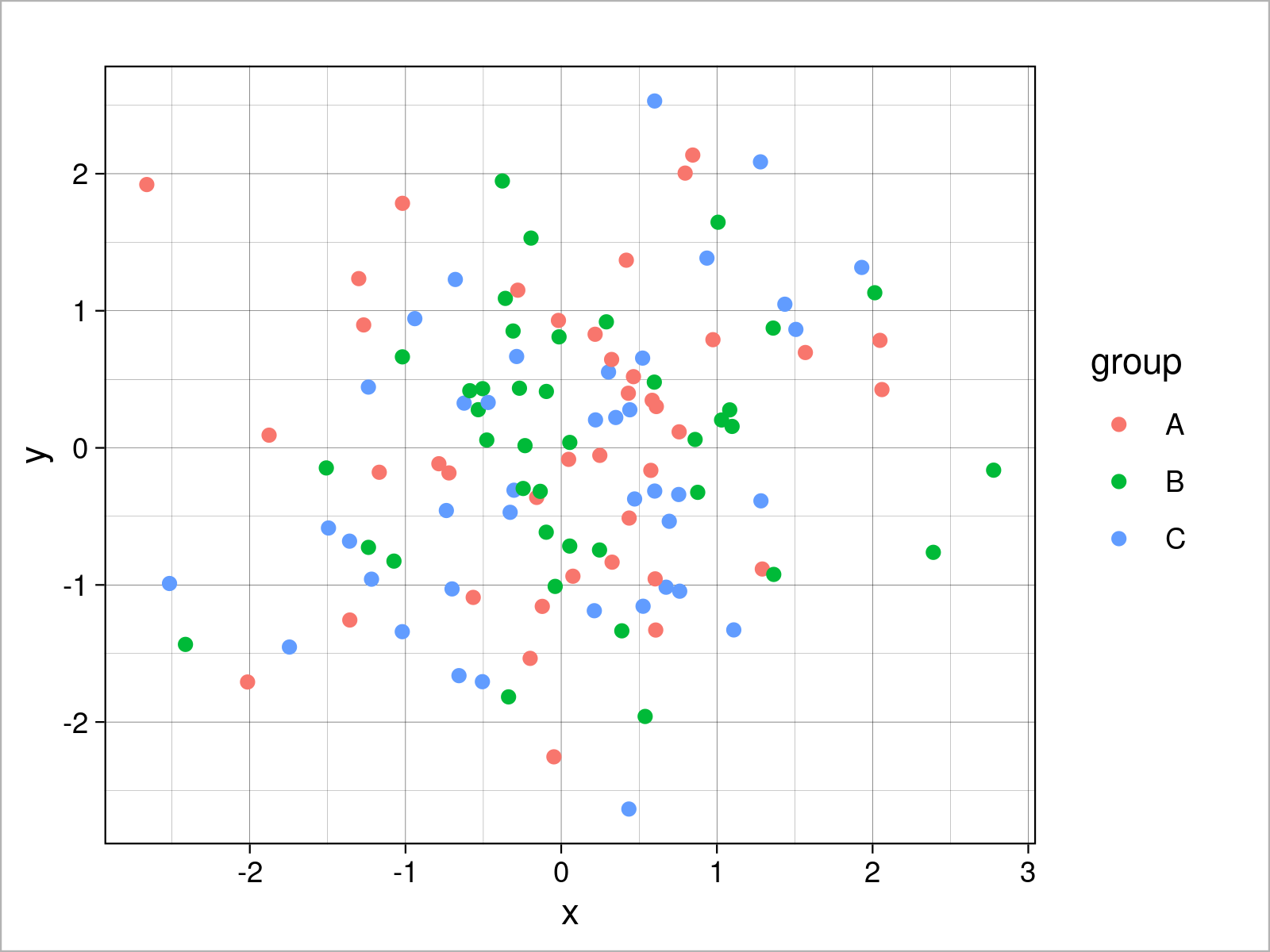# theme_linedraw ggplot2 Theme in R (6 Examples)

In this article, I’ll show how to switch the ggplot2 theme to the theme_linedraw in R.

The tutorial contains the following content:

Let’s get started!

## Example Data & Add-On Packages

The following data is used as basement for this R programming tutorial:

```set.seed(7221665)                          # Set random seed
data <- data.frame(x = rnorm(120),         # Create example data frame
y = rnorm(120),
group = LETTERS[1:3])As you can see based on Table 1, our exemplifying data is a data frame containing three columns. The variables x and y are numerical, and the variable group has the character class.

In this tutorial, I’ll also have to install and load the ggplot2 package.

```install.packages("ggplot2")                # Install ggplot2 package

## Example 1: Draw ggplot2 Scatterplot Using theme_linedraw()

```ggplot(data,                               # theme_linedraw scatterplot
aes(x = x,
y = y,
col = group)) +
geom_point() +
theme_linedraw()```## Example 2: Draw ggplot2 Density Plot Using theme_linedraw()

```ggplot(data,                               # theme_linedraw density plot
aes(x = x,
fill = group)) +
geom_density(alpha = 0.5) +
theme_linedraw()```## Example 3: Draw ggplot2 Histogram Using theme_linedraw()

```ggplot(data,                               # theme_linedraw histogram
aes(x = x,
fill = group)) +
geom_histogram(alpha = 0.5,
position = "identity",
bins = 50) +
theme_linedraw()```## Example 4: Draw ggplot2 Barchart Using theme_linedraw()

```ggplot(aggregate(y ~ group, data, sum),    # theme_linedraw barplot
aes(x = group,
y = y,
fill = group)) +
geom_bar(stat = "identity") +
theme_linedraw()```## Example 5: Draw ggplot2 Boxplot Using theme_linedraw()

```ggplot(data,                               # theme_linedraw boxplot
aes(x = x,
fill = group)) +
geom_boxplot() +
theme_linedraw()```## Example 6: Draw ggplot2 Line Plot Using theme_linedraw()

```ggplot(data,                               # theme_linedraw line plot
aes(x = x,
y = y,
col = group)) +
geom_line() +
theme_linedraw()```## Video & Further Resources

In case you want to learn more about the ggplot2 package and data visualization in R, please have a look at the following video tutorial, where I give a detailed introduction with different examples:

Please accept YouTube cookies to play this video. By accepting you will be accessing content from YouTube, a service provided by an external third party.If you accept this notice, your choice will be saved and the page will refresh.

On this website, you can also find articles on how to create different kinds of graphics such as scatterplots, density plots, barcharts, boxplots, histograms, and line plots using the other ggplot2 themes:

Furthermore, you may have a look at the introductions to other add-on packages that provide extra themes for ggplot2 plots:

Besides the video and the tutorials above, you might have a look at the other tutorials which I have published on my website.

In this R tutorial you have learned how to apply the theme_linedraw function to modify the design and layout of a ggplot2 plot. Let me know in the comments, if you have further questions.

Subscribe to the Statistics Globe Newsletter